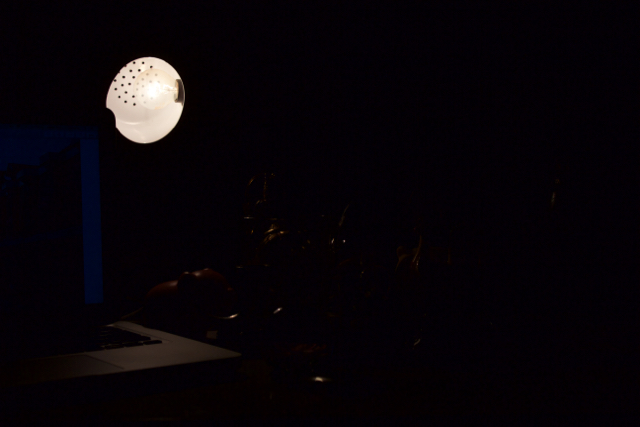This post is the fifth and final post on the series A trick you don’t know about Python: MATLAB.

In the world of Data Science and Software Development, it’s fair to say that teams are constantly getting inspiration from each other, in the form of StackOverflow responses, Reddit threads, open-source code in GitHub, research articles published on arXiv, etc. When such work reaches a certain magnitude, it is typically shared as a package, library, or toolbox (for Python, R, or MATLAB) to be used by a broad community of data scientists, developers, and engineers. However, it might be challenging to reuse it if it hasn’t been designed in your language of choice.

A friend who works in Deep Learning research using Python recently asked me how to implement High Dynamic Range Imaging, aka HDR. Though OpenCV might be the no 1. choice among Python users, this user was facing some compatibility issues with specific libraries and was looking for workarounds.

Although everyone using a smartphone might already be familiar with HDR, here is a simplified overview: it is a set of techniques that combine multiple pictures of the same scene (usually taken with different exposure times) to reproduce a greater range of luminosity than what is possible with traditional photographic techniques.

When thinking about the problem, I remembered that HDR Toolbox for MATLAB had been featured in MATLAB Central’s blog, File Exchange Pick of the Week, a few years back.

Launched in 2001, MATLAB Central is an open exchange for the MATLAB and Simulink user community. With more than 2 million visits per month, MATLAB Central hosts tens of thousands of open-source community files, answers to your programming questions, blogs offering inside views from the engineers and scientists who build and support MATLAB and Simulink, and more.

So, why not leverage the work done by the MATLAB Community and call it from Python?

The following example showcases how to do just that, for this specific example:

Credit: Francesco Banterle (2021). The HDR Toolbox. GitLab

Surely this work can be done manually. But it’s way more fun to automate it.

``````from urllib.request import urlretrieve
mltbxFilename = os.path.join(os.getcwd(),"HDRToolbox.zip")

if not os.path.exists(mltbxFilename):
urlretrieve(mltbxURL,mltbxFilename)
``````

# Install HDR Toolbox using MATLAB Engine

``````# Start MATLAB Engine
import matlab.engine
eng = matlab.engine.start_matlab("-desktop")

# Install HDR Toolbox
if not os.path.exists("HDRToolbox"):
eng.unzip(mltbxFilename, "HDRToolbox")

eng.cd("HDRToolbox",nargout=0)
eng.eval("installHDRToolbox",nargout=0)
eng.cd("..",nargout=0)
``````

# Visualize images

``````from IPython.display import Image
import os
stackFolder = os.path.join(os.getcwd(), "HDRToolbox", "demos", "stack")
stackFolderFiles = os.listdir(stackFolder)

x = []
for i in range(0, len(stackFolderFiles)):
imgFilename = os.path.join(stackFolder, stackFolderFiles[i])
x.append(Image(filename=imgFilename))
display(*x)
``````# Leverage a MATLAB-developed Community Toolbox from Python

You can easily call the MATLAB functions created by Francesco Banterle in HDR Toolbox from Python:

``````# Read a stack of LDR images
format = "jpg"

# Read exposure values from the exif

# Estimate the Camera Response Function (CRF)
linFun = eng.DebevecCRF(stack, stackExposure, 256)

# Build the radiance map using the stack and stack_exposure
imgHDR = eng.BuildHDR(stack, stackExposure, "LUT", linFun, "Deb97", "log")

# Save the radiance map in the .hdr format
outputHDR = os.path.join(stackFolder, "..", "output", "stack_hdr_image.exr")
eng.hdrimwrite(imgHDR, outputHDR, nargout=0)

# Show the tone mapped version of the radiance map with gamma encoding
fig = eng.figure("Visible", "off")
imgTMO = eng.GammaTMO(eng.ReinhardTMO(imgHDR, 0.18), 2.2, 0.0, 1.0)
outputTMO = os.path.join(stackFolder, "..", "output", "stack_hdr_image_tmo.png")
eng.imwrite(imgTMO, outputTMO, nargout=0)

Image(filename=outputTMO)

# Exit MATLAB Engine
eng.exit()
``````Using MATLAB and Python together (in either direction, MATLAB from Python or Python from MATLAB) facilitates collaboration and builds a more complete ecosystem for engineering and science.

Tags:

Categories:

Updated: